# How to draw tangent arcs between two circles in AutoCAD?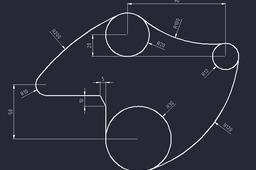In this tutorial I am going to show you the easiest way to draw arc between two circles

1. ### Step 1:Lets say we have something like this, two circles with different radius.

2. ### Step 2: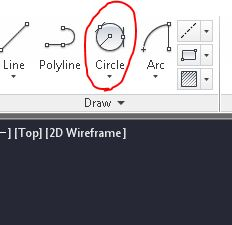If we want to draw some arc tangent to both circles with specific radius

the easiest metod (at least for me) is by using TTR (tangent tangent radius) circle.

You can find it on the circle dropdown or you can type CIRCLE and then type TTR.

3. ### Step 3: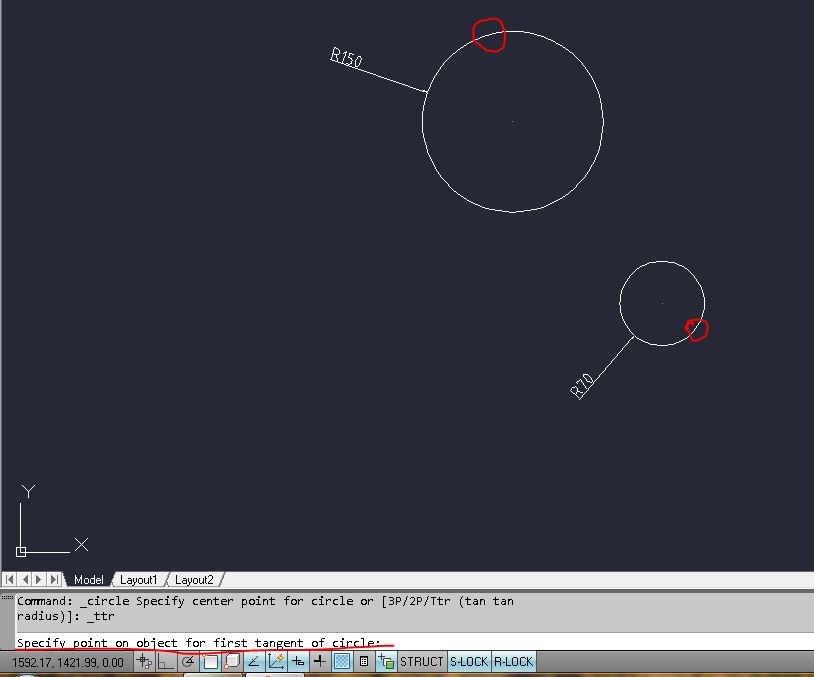So select or type the command, it will ask you: "Specify point on object for first tangent of circle" select the point on circle (red circle) but it does not matter which circle first.

4. ### Step 4:After first point it will ask you for the second and then set the radius.Mine is 400 and you should get this but only if you have radius of the two circles like mine.It does not matter if your circles have different radius, you can play with that (there are some limits though).

And by the way trim the tangent circle,I suppose you already know that :)

5. ### Step 5: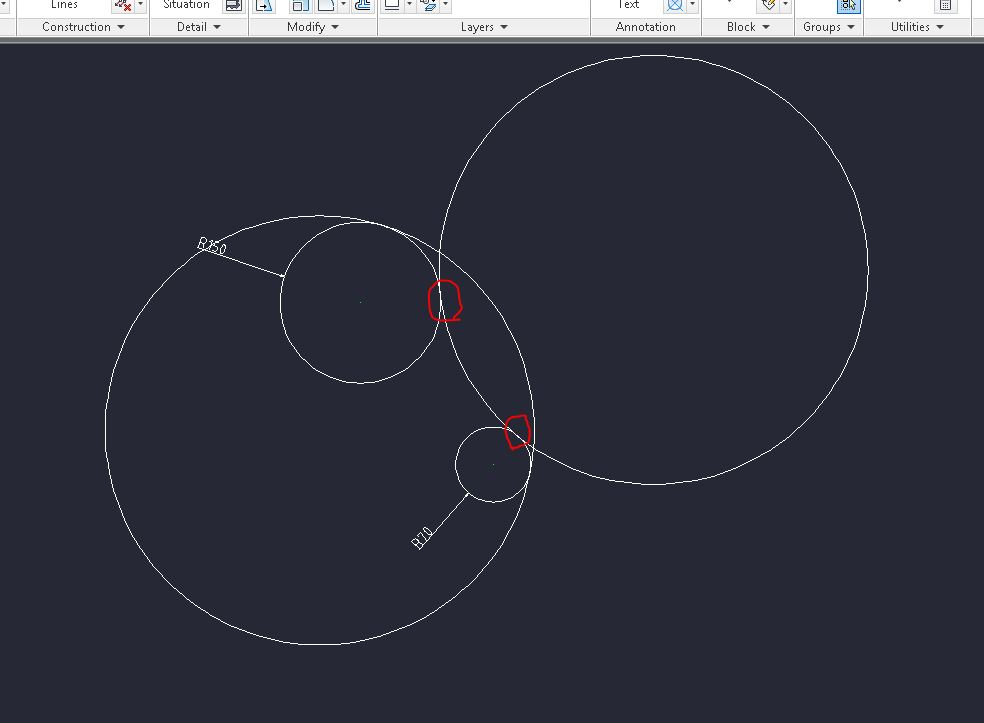Now, if you want your tangent circle to go inside select points as I checked on the picture.

6. ### Step 6: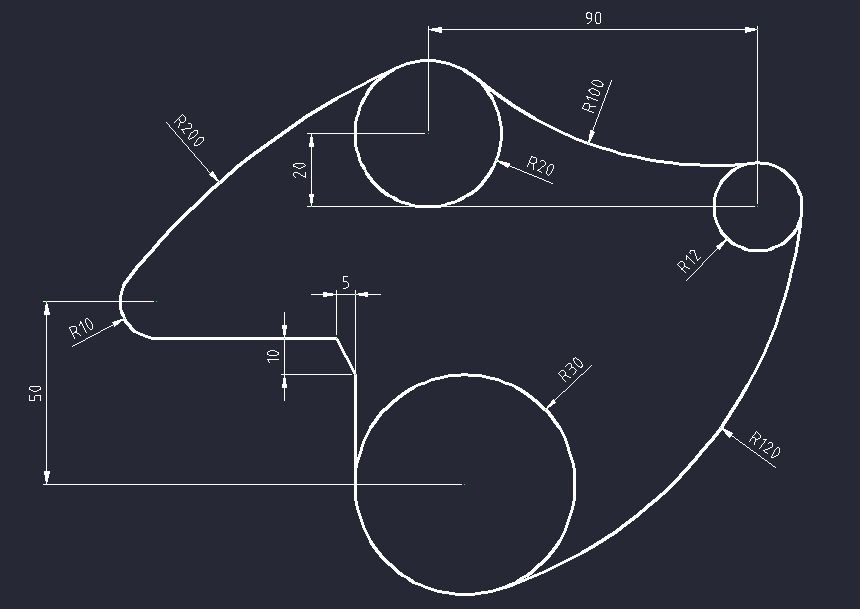That is it, here is a drawing picture for you if you wanna practice.

Note: I know that maybe this type of a tutorial is not as good as the video tutorial but I hope that somebody can learn something even from this.